Next: Multilayer perceptrons Up: Nonlinear state-space models Previous: Linear models   Contents

## Extension from linear to nonlinear

The nonlinear state-space model is in principle a very simple extension of the linear model. All that needs to be done is to replace the linear mappings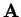and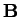of Equation (4.12) with general nonlinear mappings to get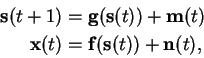(4.13)

where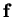and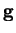are sufficiently smooth nonlinear mappings and all the other variables are as in Equation (4.12).

One of the greatest difficulties with the nonlinear model is that while a linear mapping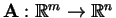can be uniquely determined by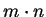real numbers (the elements of the corresponding matrix), there is no way to do the same for nonlinear mappings. Representing an arbitrary nonlinear mapping with even moderate accuracy requires much more parameters.

While the matrix form provides a natural parameterisation for linear mappings, no such evident approach exists for nonlinear mappings. There are several ways to approximate a given nonlinear mapping through different kinds of series decompositions. Unfortunately such parameterisations are often best suited for some special classes of functions or are very sensitive to the data and thus useless in the presence of noise. For example in polynomial approximations, the coefficients become very sensitive to the data when the degree of the approximating polynomial is raised. Trigonometric expansions (Fourier series) are well suited only for modelling periodic functions.

In neural network literature there are two competing nonlinear function approximation methods that are both widely used: radial-basis function (RBF) and multilayer perceptron (MLP) networks. They are both universal function approximators, meaning that given enough neurons'' they can model any function to the desired degree of accuracy .

In this work we use only MLP networks, so they are now discussed in greater detail.Next: Multilayer perceptrons Up: Nonlinear state-space models Previous: Linear models   Contents
Antti Honkela 2001-05-30